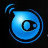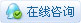##账号 自动登录 找回密码 密码 立即注册
 搜索发表于 2023-11-17 14:30:00 | 显示全部楼层 |阅读模式

### 马上注册，结交更多好友，享用更多功能，让你轻松玩转社区。

×

import { DynamicInputType, MrxDbgUiPrInt, MrxDbgUiPrPoint, MxCursorType, MxFun } from "mxdraw";

/**
* 生成规则多边形的顶点坐标
* @param {McGePoint3d} centerPoint - 多边形中心点
* @param {McGePoint3d} vertexPoint - 多边形顶点
* @param {number} sides - 多边形边数（至少为3）
* @returns {McGePoint3d[]} 多边形的顶点坐标数组
*/
export function computeRegularPolygonVertices(centerPoint = new McGePoint3d(), vertexPoint = new McGePoint3d(), sides = 3): McGePoint3d[] {
const verticesArray: McGePoint3d[] = [];
sides = Math.max(3, sides);
verticesArray.push(vertexPoint);

// 计算每个顶点的角度增量
const angleIncrement = (Math.PI * 2) / sides;

for (let i = 1; i < sides; i++) {
// 计算当前顶点对应的角度上的余弦和正弦值
const cosValue = Math.cos(angleIncrement * i),
sinValue = Math.sin(angleIncrement * i);

// 复制中心点和顶点，以免修改原始点的值
const startPt = centerPoint.clone();
const endPt = vertexPoint.clone();

// 计算相对于中心点的偏移量
const deltaX = endPt.x - startPt.x;
const deltaY = endPt.y - startPt.y;

// 根据旋转公式计算新的顶点坐标
const newX = deltaX * cosValue - deltaY * sinValue + startPt.x;
const newY = deltaX * sinValue + deltaY * cosValue + startPt.y;

// 创建新的顶点对象并加入数组
const point = new McGePoint3d(newX, newY);
verticesArray.push(point);
}
return verticesArray;
}

1.初始化参数： 首先，函数初始化了一个空数组 verticesArray 用于存储多边形的顶点坐标。同时，确保多边形的边数至少为3，如果用户输入的边数小于3，就将边数设置为3
const verticesArray: McGePoint3d[] = [];
sides = Math.max(3, sides);
verticesArray.push(vertexPoint);

2.计算角度增量： 通过将完整的圆周角（2π）除以多边形的边数，计算出每个顶点之间的角度增量。
const angleIncrement = (Math.PI * 2) / sides;

3.计算顶点坐标： 利用余弦和正弦值计算每个顶点相对于起始点的偏移量。这里采用了旋转公式，通过旋转坐标系来计算新的顶点坐标。
const cosValue = Math.cos(angleIncrement * i),
sinValue = Math.sin(angleIncrement * i);

4.复制中心点和顶点： 为了防止修改原始点的值，创建了中心点和顶点的副本。
const startPt = centerPoint.clone();
const endPt = vertexPoint.clone();

5.计算偏移量： 计算相对于中心点的偏移量，即顶点相对于中心点的位置。
const deltaX = endPt.x - startPt.x;
const deltaY = endPt.y - startPt.y;

6.旋转计算新坐标： 利用旋转公式计算新的顶点坐标，并将其添加到顶点数组中。
const newX = deltaX * cosValue - deltaY * sinValue + startPt.x;
const newY = deltaX * sinValue + deltaY * cosValue + startPt.y;
const point = new McGePoint3d(newX, newY);
verticesArray.push(point);

7.返回结果： 最终，返回计算得到的多边形的顶点坐标数组。
return verticesArray;

/**
* 计算多边形顶点坐标（基于边）
* @param {McGePoint3d} startPoint - 多边形边的起始点
* @param {McGePoint3d} endPoint - 多边形边的结束点
* @param {number} sides - 多边形边数（至少为3
* @returns {McGePoint3d[]} 多边形的顶点坐标数组
*/
export function computePolygonVerticesFromEdge(startPoint: McGePoint3d, endPoint: McGePoint3d, sides: number): McGePoint3d[] {
// 计算边的长度和角度
let dx = endPoint.x - startPoint.x;
let dy = endPoint.y - startPoint.y;
let length = Math.sqrt(dx * dx + dy * dy);
let angle = Math.atan2(dy, dx);

// 计算每个顶点的角度增量
let angleIncrement = (2 * Math.PI) / Math.max(3, sides);

let polygonVertices = [startPoint, endPoint];

for (let i = 0; i < sides; i++) {
// 计算当前顶点的坐标
let x = startPoint.x + length * Math.cos(angle + i * angleIncrement);
let y = startPoint.y + length * Math.sin(angle + i * angleIncrement);

// 更新起始点并加入数组
startPoint = new McGePoint3d(x, y);
polygonVertices.push(startPoint);
}

return polygonVertices;
}

1.计算边的长度和角度： 首先，计算给定边的长度和角度。这是通过计算起始点和结束点的横向和纵向差异，然后使用勾股定理计算长度，最后使用反正切函数计算角度。
let dx = endPoint.x - startPoint.x;
let dy = endPoint.y - startPoint.y;
let length = Math.sqrt(dx * dx + dy * dy);
let angle = Math.atan2(dy, dx);

2.计算每个顶点的角度增量： 为了均匀地分布多边形的顶点，计算每个顶点之间的角度增量。
let angleIncrement = (2 * Math.PI) / Math.max(3, sides);

3.初始化顶点数组： 创建一个数组，其中包含起始点和结束点，这是为了确保多边形是封闭的。
let polygonVertices = [startPoint, endPoint];

4.计算顶点坐标： 循环计算每个顶点的坐标。利用极坐标系的转换，通过给定的角度增量计算出每个顶点相对于起始点的坐标。
for (let i = 0; i < sides; i++) {
let x = startPoint.x + length * Math.cos(angle + i * angleIncrement);
let y = startPoint.y + length * Math.sin(angle + i * angleIncrement);
startPoint = new McGePoint3d(x, y);
polygonVertices.push(startPoint);
}

5.返回结果： 最终，返回计算得到的多边形的顶点坐标数组。
return polygonVertices;

/**
* 计算多边形顶点坐标（基于中点）
* @param {McGePoint3d} centerPoint - 多边形中心点
* @param {McGePoint3d} edgeMidPoint - 多边形一条边的中点
* @param {number} sides - 多边形边数（至少为3
* @returns {McGePoint3d[]} 多边形的顶点坐标数组
*/
function computePolygonVerticesFromMidpoint(centerPoint = new McGePoint3d(), edgeMidPoint = new McGePoint3d(), sides = 3): McGePoint3d[] {
const midX = edgeMidPoint.x;
const midY = edgeMidPoint.y;
const centerX = centerPoint.x;
const centerY = centerPoint.y;
const numberOfSides = Math.max(3, sides);

// 计算中点到多边形中心的距离
const distanceToCenter = Math.sqrt((midX - centerX) ** 2 + (midY - centerY) ** 2);

// 计算中点到多边形中心的半径
const radius = distanceToCenter / Math.cos(Math.PI / numberOfSides);

// 计算起始角度
const startAngle = Math.atan2(midY - centerY, midX - centerX) - Math.PI / numberOfSides;

const vertices = [];

for (let i = 0; i < numberOfSides; i++) {
// 计算当前顶点的角度
const angle = startAngle + (i * 2 * Math.PI / numberOfSides);

// 根据极坐标系转换成直角坐标系的坐标
const x = centerX + radius * Math.cos(angle);
const y = centerY + radius * Math.sin(angle);

// 创建新的顶点对象并加入数组
vertices.push(new McGePoint3d(x, y));
}

return vertices;
}

1.获取中点和中心点的坐标： 首先，获取给定边的中点（edgeMidPoint）和多边形的中心点（centerPoint）的坐标。
const midX = edgeMidPoint.x;
const midY = edgeMidPoint.y;
const centerX = centerPoint.x;
const centerY = centerPoint.y;

2.计算中点到中心的距离和半径： 利用勾股定理计算中点到中心的距离，然后计算出多边形的半径。
const distanceToCenter = Math.sqrt((midX - centerX) ** 2 + (midY - centerY) ** 2);
const radius = distanceToCenter / Math.cos(Math.PI / numberOfSides);

3.计算起始角度： 利用反正切函数计算出中点到中心的方向角，并减去角度增量的一半，以确保多边形顶点的均匀分布。
const startAngle = Math.atan2(midY - centerY, midX - centerX) - Math.PI / numberOfSides;

4.计算顶点坐标： 循环计算每个顶点的坐标。通过极坐标系转换，将极坐标系中的角度转换为直角坐标系中的坐标。
for (let i = 0; i < numberOfSides; i++) {
const angle = startAngle + (i * 2 * Math.PI / numberOfSides);
const x = centerX + radius * Math.cos(angle);
const y = centerY + radius * Math.sin(angle);
vertices.push(new McGePoint3d(x, y));
}

5.返回结果： 最终，返回计算得到的多边形的顶点坐标数组。
return vertices;

/**
* 绘制多边形的函数
*/
export async function drawPolygon() {
// 创建用户输入对象，用于获取侧面数
getNum.setMessage("\n输入侧面数 <5>")

// 获取用户输入的侧面数
let sideNum = await getNum.go() as number;
if (!sideNum) sideNum = 5;

// 创建用户输入对象，用于获取多边形的中心点或边
getPoint.setMessage("\n指定正多变形的中心点");
getPoint.setKeyWords("[(E)]");

// 设置光标类型
getPoint.setCursorType(MxCursorType.kCross);

// 获取用户输入的中心点或边
const centerPoint = await getPoint.go();

if (!centerPoint) {
// 如果用户选择边，进入边绘制流程
if (getPoint.isKeyWordPicked("e")) {
// 获取用户输入的边的第一个端点
getPoint.setMessage("\n指定边的第一个端点");
getPoint.setKeyWords("");
const startPoint = await getPoint.go();

if (!startPoint) return;

// 设置用户绘制回调函数，用于实时绘制多边形
getPoint.setUserDraw((currentPoint, pWorldDraw) => {
const pPolyline = new McDbPolyline();
// 计算多边形顶点
const points = computePolygonVerticesFromEdge(startPoint, currentPoint, sideNum);

// 将顶点添加到多边形
points.forEach((point) => {
});

// 设置多边形为闭合状态
pPolyline.isClosed = true;

// 实时绘制多边形
pWorldDraw.drawMcDbEntity(pPolyline);
});

// 获取用户输入的边的第二个端点
getPoint.setMessage("\n指定边的第二个端点");
await getPoint.go();

// 绘制多边形并清除绘制保留
getPoint.drawReserve();
}
return;
}

// 用户选择中心点后的绘制流程
getPoint.setMessage("\n输入选项");
getPoint.setKeyWords("[内接于圆(I)/外切于圆(C)]");

// 获取用户选择的是内切圆还是外切圆
await getPoint.go();
let isTangentToTheCircle = true;
if(getPoint.isKeyWordPicked("i")) isTangentToTheCircle = false;

// 设置用户绘制回调函数，用于实时绘制多边形
getPoint.setUserDraw((currentPoint, pWorldDraw) => {
// 获取当前绘图颜色

// 创建多边形对象
const pPolyline = new McDbPolyline();
pPolyline.trueColor = new McCmColor(drawColor.r, drawColor.g, drawColor.b);

// 计算多边形顶点
const points = isTangentToTheCircle ? computePolygonVerticesFromMidpoint(centerPoint, currentPoint, sideNum) : computeRegularPolygonVertices(centerPoint, currentPoint, sideNum);

// 将顶点添加到多边形
points.forEach((pt) => {
});

// 设置多边形为闭合状态
pPolyline.isClosed = true;

// 实时绘制多边形
pWorldDraw.drawMcDbEntity(pPolyline);
});

// 获取用户输入的圆的半径
getPoint.setMessage("\n指定圆的半径");
await getPoint.go();

// 绘制多边形并清除绘制保留
getPoint.drawReserve();
}

1.用户输入

getNum.setMessage("\n输入侧面数 <5>")
let sideNum = await getNum.go() as number;
if (!sideNum) sideNum = 5;

getPoint.setMessage("\n指定正多变形的中心点");
getPoint.setKeyWords("[(E)]");

2.选择中心点或边

const centerPoint = await getPoint.go();
if (!centerPoint) {
if (getPoint.isKeyWordPicked("e")) {
// 边绘制流程...
}
return;
}

3.边绘制流程

const startPoint = await getPoint.go();
if (!startPoint) return;

getPoint.setUserDraw((currentPoint, pWorldDraw) => {
// 实时绘制多边形...
});

await getPoint.go();
getPoint.drawReserve();

4.中心点绘制流程

getPoint.setMessage("\n输入选项");
getPoint.setKeyWords("[内接于圆(I)/外切于圆(C)]");
await getPoint.go();

getPoint.setUserDraw((currentPoint, pWorldDraw) => {
// 实时绘制多边形...
});

getPoint.setMessage("\n指定圆的半径");
await getPoint.go();
getPoint.drawReserve();

5.实时绘制多边形

getPoint.setUserDraw((currentPoint, pWorldDraw) => {
// 获取当前绘图颜色...

// 创建多边形对象...
const pPolyline = new McDbPolyline();
pPolyline.trueColor = new McCmColor(drawColor.r, drawColor.g, drawColor.b);

// 计算多边形顶点...
const points = isTangentToTheCircle ? computePolygonVerticesFromMidpoint(centerPoint, currentPoint, sideNum) : computeRegularPolygonVertices(centerPoint, currentPoint, sideNum);

// 将顶点添加到多边形...
points.forEach((pt) => {
});

// 设置多边形为闭合状态...
pPolyline.isClosed = true;

// 实时绘制多边形...
pWorldDraw.drawMcDbEntity(pPolyline);
});

### 评分XDSoft + 20 + 5 很给力!经验；技术要点；资料分享奖！.

 本版积分规则 回帖并转播 回帖后跳转到最后一页|申请友链|Archiver|手机版|小黑屋|辽公网安备|晓东CAD家园 ( 辽ICP备15016793号 )

GMT+8, 2023-12-4 18:26 , Processed in 0.375707 second(s), 28 queries , Gzip On.